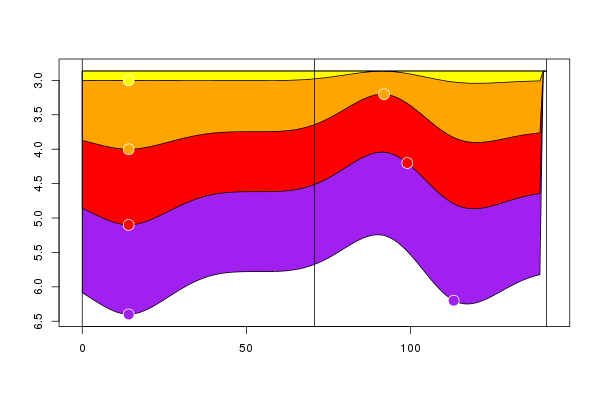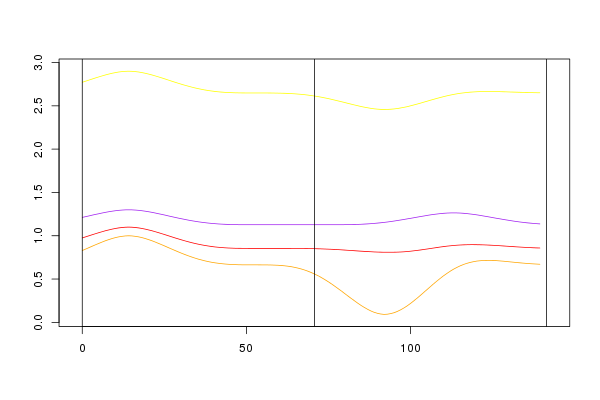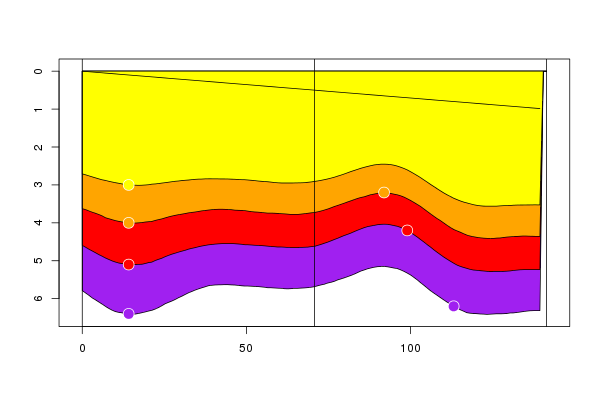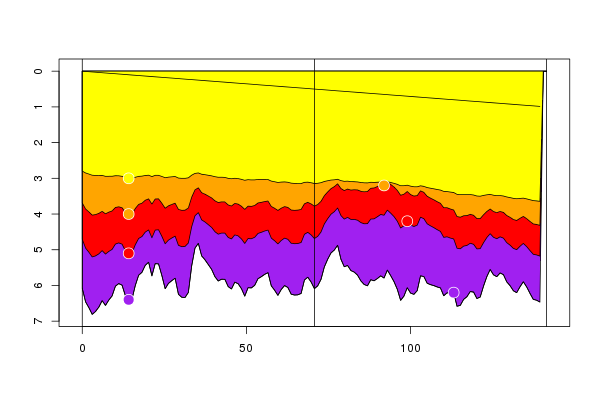How to perform a Multi-Layer estimation

How to use RGeostats package step-by-step.

How to perform a Multi-Layer estimation

This paragraph illustrates the methodology (initially developped within the package Isatoil) which enables a joint estimation of a set of surfaces in a layer-cake framework. This methodology is based on a multi-variate approach (as many variables as they are surfaces).
It is based on the information provided by the intercepts of well data with those surfaces. The spatial characteristics of the surfaces are captured in a multivariate model defined in covariances. This technique enables dealing with any kind of well information (vertical, inclined, deviated or horizontal) as it relies on the joint estimation of cumulative layer thicknesses (True Vertical Depth).

The method offers some interesting properties, such as:
* to define the reference surface which serves as a support to the whole layer cake system
* to establish the spatial multivariate model either on thicknesses or on velocities (if exhaustive time maps are provided). The results are then produced either in cumulative thickness maps or in layer velocity maps
* to constraint the whole layer-cake package not to exceed a bottom map (specified exhaustively)

This case study performs the different types of multilayer estimations with different hypotheses. All the estimations are represented through a vertical section across the field.

Defining the environment
The first task is to define the well information: this consists in the intercepts with the different surfaces. Data are characterized by their 2-D coordinates, the elevation of the intercept and the rank of the intercepted variable (locator: layer). The total number of surfaces is equal to 4. The following paragraph describes the contents of the ASCII data file.
Code: Select all
`x1 x2 z1 layer10. 10.  3.   110. 10.  4.   210. 10.  5.1  310. 10.  6.4  480. 10.  3.5  181. 9.5  3.8  281. 9.2  5.5  412. 75.  2.6  112. 75.  3.8  212. 75.1 4.5  312. 75.4 5.5  465. 65.  3.2  270. 70.  4.2  380. 80.  6.2  4`

A second part of the environment consists in the set of 4 colors used to represent the layers. This color scale will be stored in the local variable colors.
Code: Select all
`colors = c("yellow","orange","red","purple")`

Creating the data bases
The first task is to read the ASCII file containing the data (called data.ascii in this paragraph) and to load them in db. Moreover a 2-D grid is created covaring the area of interest. A trace (stored in the object trace) is defined which crosses the field along its first diagonal.
Finally all the case study will be performed using a Unique Neighborhood which is created here and saved in the object called neigh.
Code: Select all
`db = db.create(read.table("a.dat",header=T))grid = db.create(nx=c(100,100))trace = matrix(c(0,0,50,50,100,100),ncol=2,byrow=TRUE)neigh = neigh.init(ndim=2,type=0)`

Several variables are created in the Grid file which will be used to demonstrate some of the options:

1) a surface is generated as a tilted plane covering the field: it will possibily be used as the reference surface (hence its name):
Code: Select all
`grid = db.add(grid,reference=(x1+x2)/200)`

2) a more irregular surface is generated which will possibly be used as the bottom surface (hence its name). This surface is simulated using a simulation (with a short range spherical model), conditioned by the well intercepts with the layer #4:
Code: Select all
`model = model.create(vartype="Spherical",range=10,sill=0.2)db   = db.sel(db,layer==4)grid = simtub(db,grid,model,neigh,seed=0)grid = db.rename(grid,5,"bottom")db   = db.delete(db,"sel")rm(model)`

3) a set of 4 time surfaces are generated: they are simulated unconditionally in the time system, with different means (1000m/s for the first one, 1200 for the second one, 1400 for the third one and 1600 for the last one). For simplicity, they all follow the same spatial characteristics: short range spherical model with the same variance:
Code: Select all
`model = model.create(vartype="Spherical",range=10,sill=4)grid = simtub(,grid,model,uc=NULL,mean=1000,seed=0)grid = simtub(,grid,model,uc=NULL,mean=1200,seed=0)grid = simtub(,grid,model,uc=NULL,mean=1400,seed=0)grid = simtub(,grid,model,uc=NULL,mean=1600,seed=0)grid = db.locate(grid,seq(6,9),"time")rm(model)`

The multivariate model used in all subsequent interpolations is defined here. For simplicity sake, all the variables are independent and follow the same spatial characteristics (with a cubic variogram) with different sills:
Code: Select all
`sill  = matrix(rep(0,4*4),nrow=4,ncol=4)diag(sill) = c(1,3,2,4)model = model.create(vartype="Cubic",range=40,sill=sill)`

Multilayers estimation
It is now time to perform the joint estimation of the set of surfaces of the layer cake. For all the different options, we store the results in an auxiliary Grid file (called a) and display the them along the vertical section (with the vertical axis oriented downwards).

The first attempt is the standard interpolation when defining no reference not bottom surfaces.
Code: Select all
`a = mlayer.krig(db,grid,model,neigh,flag.std=FALSE)db.section.plot(trace,a,db,cex=2,pch=21,fg.col="white",cols=colors,flag.up=FALSE)`

and the corresponding section:In the following case, a reference surface is added and the same interpolation is carried on.
Code: Select all
`a = mlayer.krig(db,grid,model,neigh,colrefd="reference")db.section.plot(trace,a,db,addnames="reference",flag.up=FALSE,cex=2,pch=21,fg.col="white",cols=colors)`

which leads to the following section:The results of the previous attempt are presented in layer thickness. Obviously it does not make sense to overlay the well intercepts provided in depth.
Code: Select all
`a = mlayer.krig(db,grid,model,neigh,colrefd="reference",flag.z=FALSE)db.section.plot(trace,a,flag.fill=FALSE,cex=2,pch=21,fg.col="white",cols=colors)`

The section is now produced in thickness scale:The same interpolation can be performed considering that the spatial characteristics correspond to the velocities (rather than to thicknesses):
Code: Select all
`a = mlayer.krig(db,grid,model,neigh,flag.vel=TRUE,colrefd="reference")db.section.plot(trace,a,db,addnamesl="reference",flag.up=FALSE,cex=2,pch=21,fg.col="white",cols=colors)`

The resulting section is displayed next:This last trial produces the layer velocities obtained in the same circonstances:
Code: Select all
`a = mlayer.krig(db,grid,model,neigh,flag.vel=TRUE,flag.z=FALSE,colrefd="reference")db.section.plot(trace,a,flag.fill=FALSE,cex=2,pch=21,fg.col="white",cols=colors)`

The section represents the velocities:In this last trial, we define both the reference and the bottom surfaces and perform the interpolation in depth. These two external surfaces are also displayed.
Code: Select all
`a = mlayer.krig(db,grid,model,neigh,colrefd="reference",colrefb="bottom")db.section.plot(trace,a,db,addnames=c("reference","bottom"),flag.up=FALSE,   cex=2,pch=21,fg.col="white",cols=colors)`

The corresponding section is presented next:Didier Renard

Posts: 289
Joined: Thu Sep 20, 2012 4:22 pm